#### 期刊菜单

Large Deformation of a Rod with Compress and Bending Moment Load Using Accurate Geometric Model
DOI: 10.12677/IJM.2022.111001, PDF, HTML, XML, 下载: 75  浏览: 160  国家自然科学基金支持

Abstract: Based on accurate geometric model, the large deformation of a rod with free-fixed edges subjected to compress and bending moment loads was studied. Considering the precise geometric relation-ships across the deformation process, the geometric equations and equilibrium equations were es-tablished, and the basis equations of exact geometry under large deformation with linear constitu-tive relationship were established. Using shooting method to numerically solve the boundary value problem, the exact solution in the numerical sense was obtained, and the characteristic figures of the elastic large deformation with compress and bending moment loads were drawn, the influence of the large deformation elastic rod with external load was studied, and the mechanical characteris-tics of the rod were analyzed.

1. 引言

1744年Euler等  研究了弹性细杆的平衡和稳定性问题。1927年Love等  进一步研究了细长杆的弹性曲线问题，细长弹性杆的几何非线性问题作为结构大变形的问题之一始终受到广泛重视。Kicrhhoff理论(即弹性杆静力学非线性理论)建立于1859年。Kicrhhoff理论其实质为利用欧拉-泊松(Euler-Poison)方程以及弹性杆平衡微分方程两者在数学形式中的相似性，将拉格朗日情形刚体定点转动的解析积分进行移植，以用来解决弹性杆的平衡问题 。Pflǜger  是第一位研究压杆在轴向压缩变形下对屈曲变形影响的人。Antanackvic  等采用了分析方法研究在端部压力作用下可伸长简支梁过屈曲的分叉解，并得出了和细长比相关的临界载荷解析式。Gummadi和Palazotto  对梁、拱中的大变形问题进行了研究，对现代大变形力学问题的研究产生了极大的影响。基于连续介质一般理论，Filipich和Rosales   系统推导出弹性细杆在轴向压力作用下的几何精确非线性控制方程，推导出了弹性压杆在9种本构关系下的过屈曲模型以及数值解，并研究在轴向载荷作用下弹性杆本构关系不同时对过屈曲行为的影响。Plaut等  基于弹性杆的轴线不可伸长几何非线性理论，采用打靶法对问题数值求解，分析了在外载荷作用下预弯曲杆在与刚性平面发生接触后的屈曲和变形问题。

2. 控制方程

2.1. 几何方程

$\frac{\text{d}s}{\text{d}x}=\lambda$ (1)

$\frac{\text{d}u}{\text{d}x}=\lambda \mathrm{cos}\theta -1$ (2)

$\frac{\text{d}w}{\text{d}x}=\lambda \text{sin}\theta$ (3)

$\frac{\text{d}\theta }{\text{d}x}=\frac{\text{d}\theta }{\text{d}s}×\frac{\text{d}s}{\text{d}x}=\lambda \frac{1}{\rho }=\lambda k$ (4)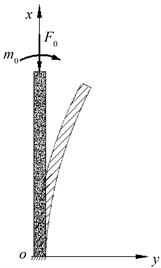Figure 1. Mechanical model of structure

${\epsilon }_{0}=\frac{\text{d}s-\text{d}x}{\text{d}x}=\lambda -1$

$\epsilon =\frac{\text{d}{s}^{\prime }-\text{d}x}{\text{d}x}=\frac{\text{d}\theta \left(\rho -y\right)}{\text{d}x}-1=\lambda -1-y\lambda k$

2.2. 物理方程

$\sigma =E\epsilon$

${F}_{N}={\int }_{-h/2}^{h/2}\sigma b\text{d}y=EA\left(\lambda -1\right)$

$m={\int }_{-h/2}^{h/2}-\sigma by\text{d}y=EI\lambda k$

2.3. 平衡方程

${F}_{N}={F}_{v}\mathrm{cos}\theta ,\text{}{F}_{S}={F}_{v}\mathrm{sin}\theta$

$\frac{\text{d}{F}_{N}}{\text{d}x}=-{F}_{v}\mathrm{sin}\theta \frac{\text{d}\theta }{\text{d}x}=-{F}_{N}\lambda k\mathrm{tan}\theta$

$\frac{\text{d}m}{\text{d}x}={F}_{v}\frac{\text{d}w}{\text{d}x}={F}_{N}\lambda \mathrm{tan}\theta$

$\frac{\text{d}\lambda }{\text{d}x}=-\left(\lambda -1\right)\lambda k\mathrm{tan}\theta$ (5)

$\frac{\text{d}k}{\text{d}x}=\left(\lambda -1\right){k}^{2}\mathrm{tan}\theta +\frac{\left(\lambda -1\right)A\mathrm{tan}\theta }{I}$ (6)

$固定端\left(x=0\right):u=w=\theta =s=0$ (7)

$自由端\left(x=l\right):{F}_{v}=-{F}_{0},m={m}_{0}$ (8)

3. 对控制方程无量纲化

$\left(X,S,U,W\right)=\left(x,s,u,w\right)/l$

$K=k\cdot l,\text{}Τ=\theta ,\text{}\Lambda =\lambda ,\text{}\delta =h/l$

$N={F}_{N}/EA{\delta }^{2},\text{}M=ml/EI\delta$

${V}_{0}={F}_{0}/EA{\delta }^{2},\text{}{M}_{0}={m}_{0}l/EI\delta$

$N=\left(\Lambda -1\right)/{\delta }^{2},\text{}M=\Lambda K/\delta$ (9)

$\frac{\text{d}S}{\text{d}X}=\Lambda$ (10)

$\frac{\text{d}Τ}{\text{d}X}=\Lambda K$ (11)

$\frac{\text{d}U}{\text{d}X}=\Lambda \mathrm{cos}Τ-1$ (12)

$\frac{\text{d}W}{\text{d}X}=\Lambda \text{sin}Τ$ (13)

$\frac{\text{d}\Lambda }{\text{d}X}=-\left(\Lambda -1\right)\Lambda K\mathrm{tan}Τ$ (14)

$\frac{\text{d}K}{\text{d}X}=\left(\Lambda -1\right){K}^{2}\mathrm{tan}Τ+\frac{\left(\Lambda -1\right)A\mathrm{tan}Τ}{I}$ (15)

$固定端\left(x=0\right):S=Τ=U=W=0$ (16)

$自由端\left(x=1\right):\left(\Lambda -1\right)+{V}_{0}{\delta }^{2}\mathrm{cos}Τ=0,\text{}\Lambda K-{M}_{0}\delta =0$ (17)

4. 弯曲问题的数值分析

$Y={\left\{{y}_{1},{y}_{2},{y}_{3},{y}_{4},{y}_{5},{y}_{6}\right\}}^{\text{T}}={\left\{S,U,W,Τ,\Lambda ,K\right\}}^{\text{T}}$ (18)

$\frac{\text{d}Y}{\text{d}X}=F\left(X;Y;\delta ,{V}_{0},{M}_{0}\right)=\left\{{y}_{5},U,W,Τ,\Lambda ,K\right\}$ (19)

$\frac{\text{d}{y}_{1}}{\text{d}x}={y}_{5},\text{}\frac{\text{d}{y}_{2}}{\text{d}x}={y}_{5}\cdot \mathrm{cos}\left({y}_{4}\right)-1,\text{}\frac{\text{d}{y}_{3}}{\text{d}x}={y}_{5}\cdot \mathrm{sin}\left({y}_{4}\right),\text{}\frac{\text{d}{y}_{4}}{\text{d}x}={y}_{5}\cdot {y}_{6}$ (20)

$\frac{\text{d}{y}_{5}}{\text{d}x}={y}_{6}\cdot {y}_{5}\cdot \left(1-{y}_{5}\right)\cdot \mathrm{tan}\left({y}_{4}\right),\text{}\frac{\text{d}{y}_{6}}{\text{d}x}=\left({\delta }^{2}\cdot {y}_{6}{}^{2}+12\right)\cdot \left(1-{y}_{5}\right)\cdot \mathrm{tan}\left({y}_{4}\right)/{\delta }^{2}$ (21)

$固定端:{y}_{1}=0,{y}_{2}=0,{y}_{3}=0,{y}_{4}=0$ (22)

$自由端:\left({y}_{5}-1\right)-{V}_{0}\cdot \text{cos}\left({y}_{4}\right)\cdot {\delta }^{2}=0,\text{}{y}_{5}\cdot {y}_{6}-{M}_{0}\cdot \delta =0$ (23)

${V}_{cr}=\frac{{\pi }^{2}EI}{{\left(\mu l\right)}^{2}}\cdot \frac{1}{EA{\delta }^{2}}=0.\text{2}0\text{56}$

${M}_{0}=0$ 时， ${V}_{0}$ 达到临界载荷前，压杆的轴向位移几乎为0，而达到临界载荷后，轴向位移明显增加，变形从轴向压缩变形过渡到过屈曲变形。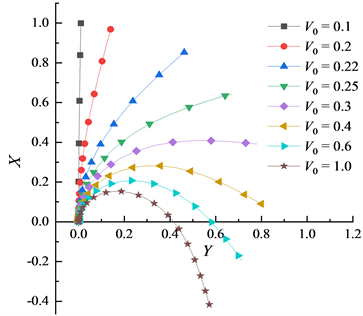Figure 2. Equilibrium configurations at ${M}_{0}=0.1$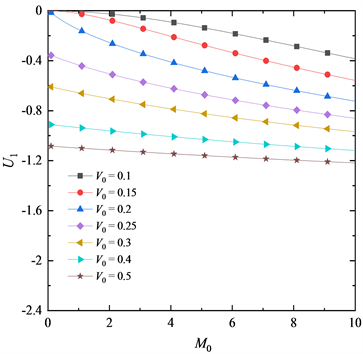Figure 3. Variation curves of ${U}_{1}$ with ${M}_{0}$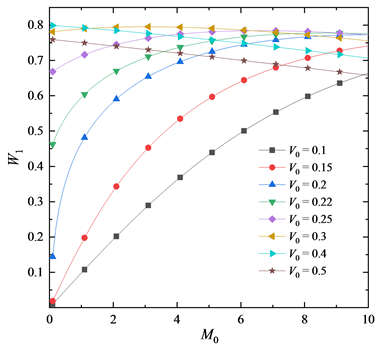Figure 4. Variation curves of ${W}_{1}$ with ${M}_{0}$Figure 5. Variation curves of ${S}_{1}$ with ${M}_{0}$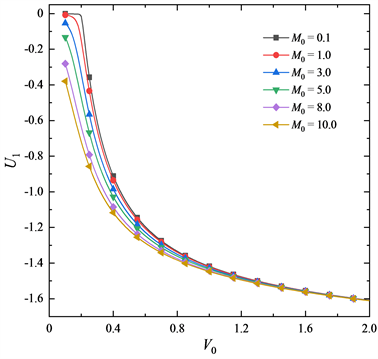Figure 6. Variation curves of ${U}_{1}$ with ${V}_{0}$Figure 7. Variation curves of ${W}_{1}$ with ${V}_{0}$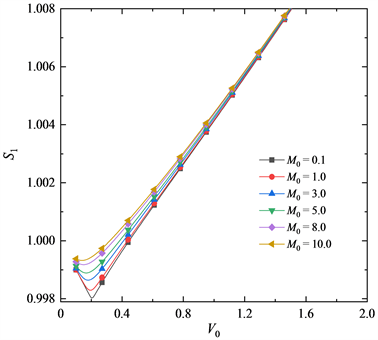Figure 8. Variation curves of ${S}_{1}$ with ${V}_{0}$

5. 结论

1) 本文基本方程采用了大变形下精确的几何模型，既可求解压杆的过屈曲问题，也可精确求解杆件的大变形弯曲问题。

2) 由精确几何模型下的基本方程求得的小变形时的数值结果与参考文献中的结果基本一致，而本文的基本方程更为精确。

3) 弹性杆压弯变形时，杆件变形随 ${M}_{0}$ 的变化规律受到 ${V}_{0}$ 的影响，在 ${M}_{0}$ 较小时， ${V}_{0}$ 的影响比较明显。同时杆件变形随 ${V}_{0}$ 的变化规律也受到 ${M}_{0}$ 的影响，当在 ${V}_{0}$ 临界载荷附近以及小于临界载荷时， ${M}_{0}$ 对变形的影响较为明显；随 ${V}_{0}$ 的增大， ${M}_{0}$ 对变形的影响逐渐减弱。

  刘延柱. 弹性杆基因模型的力学问题[J]. 力学与实践, 2003(1): 1-5.  刘延柱. 超大变形弹性细杆几何形态的拓扑描述[J]. 力学与实践, 2004, 26(5): 68-70.  Euler, L. (1744) Methodus Inveniendi Lineas Curvas Maximi Minimive Proprietate Gaudentes.  Love, A.E.H. (1927) A Treatise on the Mathematical Theory of Elasticity. Dover, New York.  Lagrange, J.L. (1970) Oeuvres de Lagrange. Readex Microprint, New York.  Pfluger, A. (1964) Stabilitäts probleme der Elastostatik. Springer, Berlin. https://doi.org/10.1007/978-3-662-25023-5  Atanackovic, T.M. (1989) Stability of a Compressible Elastic Rod with Imperfections. Acta Mechania, 76, 203-222. https://doi.org/10.1007/BF01253580  Gummadi, L. and Palazotto, A.N. (1998) Large Strain Analysis of Beams and Arches Undergoing Large Rotations. International Journal of Non-Linear Mechanics, 33, 615-645. https://doi.org/10.1016/S0020-7462(97)00033-4  Filpich, C.P. and Rosales, M.B. (1994) Contribution to the Study of the Static Postbuckling of Elastic Columns. Proceedings of the XVI Jornadas Argentinas de Ingeniería Estruc-tural, Vol. I, 197-212.  Filpich, C.P. and Rosales, M.B. (2000) A Further Study on the Postbuckling of Extensible Elastic Rods. International Journal of Non-Linear Mechanics, 35, 997-1022. https://doi.org/10.1016/S0020-7462(99)00076-1  Plaut, R.H., Suherman, S., et al. (1999) Deflections and Buckling of a Bent Elastica in Contact with a Flat Surface. International Journal of Solids & Structures, 36, 1209-1229. https://doi.org/10.1016/S0020-7683(97)00348-X  薛纭, 翁德玮. 轴线存在应变时弹性杆力学的两个概念[J]. 力学与实践, 2011, 33(5): 65-67.  刘延柱, 薛纭, 等. 关于弹性梁的数学模型[J]. 力学与实践, 2011, 33(1): 74-77.  李世荣, 李中明. 压杆过屈曲分析中轴线无伸长假设的定量讨论[J]. 兰州大学学报: 自然科学版, 1997, 33(4): 42-46.  李世荣, 杨静宁. 可伸长变截面杆的弹性过屈曲模型及其数值解[J]. 计算力学学报, 2000, 17(1): 114-118.  Li, S.R. and Batra, R.C. (2007) Thermal Buckling and Postbuckling of Euler Bernoulli Beams Supported on Nonlinear Elastic Foundations. AIAA Journal, 45, 712-720. https://doi.org/10.2514/1.24720  郭锐, 李世荣, 张靖华. 点间隙约束下轴向受压弹性梁的过屈曲[J]. 甘肃科学学报, 2010, 22(4): 96-99.  李银山, 刘波, 潘文波, 等. 弹性压杆的大变形分析[J]. 河北工业大学学报, 2011, 40(5): 31-35.  薛纭, 王鹏. 弹性细杆弯扭度有突变时的Lagrange方程[J]. 动力学与控制学报, 2019, 17(5): 473-477.  刘荣刚, 边文凤, 李素超, 刘伟, 金松波. 理想弹性杆临界挠度的确定[J]. 力学与实践, 2020, 42(4): 116-118.